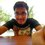# Polynomial Doubt

Question :

1. $x^4 + px^3 + qx^2 + px + 1 =0$ has real roots. Then what is the minimum value of $p^2 +q^2$ .

How I started ?
I started by dividing the whole equation by $x^2$ then we get
$(x + \frac{1}{x} ) ^2 + p (x + \frac{1}{x} ) + q - 2 = 0$
Then put $(x + \frac{1}{x} ) = t$. Then discriminant should be greater than equal to zero. But now the problem arises that t does not belong to (-2,2) , so taking care of that part leads to solving inequality which I am unable to do .

Have I started the right way?
One more thing to notice is that the sum of roots of the equation is equal to the sum of reciprocal of the roots .
How to proceed further ?Note by Anurag Pandey
4 years, 6 months ago

This discussion board is a place to discuss our Daily Challenges and the math and science related to those challenges. Explanations are more than just a solution — they should explain the steps and thinking strategies that you used to obtain the solution. Comments should further the discussion of math and science.

When posting on Brilliant:

• Use the emojis to react to an explanation, whether you're congratulating a job well done , or just really confused .
• Ask specific questions about the challenge or the steps in somebody's explanation. Well-posed questions can add a lot to the discussion, but posting "I don't understand!" doesn't help anyone.
• Try to contribute something new to the discussion, whether it is an extension, generalization or other idea related to the challenge.

MarkdownAppears as
*italics* or _italics_ italics
**bold** or __bold__ bold
- bulleted- list
• bulleted
• list
1. numbered2. list
1. numbered
2. list
Note: you must add a full line of space before and after lists for them to show up correctly
paragraph 1paragraph 2

paragraph 1

paragraph 2

[example link](https://brilliant.org)example link
> This is a quote
This is a quote
    # I indented these lines
# 4 spaces, and now they show
# up as a code block.

print "hello world"
# I indented these lines
# 4 spaces, and now they show
# up as a code block.

print "hello world"
MathAppears as
Remember to wrap math in $$ ... $$ or $ ... $ to ensure proper formatting.
2 \times 3 $2 \times 3$
2^{34} $2^{34}$
a_{i-1} $a_{i-1}$
\frac{2}{3} $\frac{2}{3}$
\sqrt{2} $\sqrt{2}$
\sum_{i=1}^3 $\sum_{i=1}^3$
\sin \theta $\sin \theta$
\boxed{123} $\boxed{123}$

Sort by:

Great start! So what you have is: The quadratic equation $t^2 + pt + q-2 = 0$ must have 2 roots that are not in $(-2,2)$. What are the necessary and sufficient condtions for this to happen?

For example, you stated that "discriminant must be positive/non-negative", which is clearly necessary, but not sufficient. How can we check to ensure that the roots are in the desired regions?

Staff - 4 years, 6 months ago

As the coefficient of ' t ' is positive so the value of the quadratic at x=-2 ans at x =2 must be less than or equal to zero. Equality can hold as it can take value -2 and 2. Is this condition sufficient ?

- 4 years, 6 months ago

No necessarily. $( x - 100)^2$ satisfies the requirements, but the value at 2, -2 is positive.

Think through all the possible cases and their implications.

Staff - 4 years, 6 months ago

Okay . I forgot to take in account the case where discriminant is equal to zero. In that case the value of quadratic at $x=\pm{2}$ will be greater than or equal to zero .

- 4 years, 6 months ago

@Calvin Lin Thank you Sir !

- 4 years, 6 months ago

Staff - 4 years, 6 months ago

Hey, can you help me in solving the case when p^2>4(q-2)? How do we obtain the minimum value for this case?

- 4 years, 6 months ago

$p^2 > 4(q-2)$ has to be true for the quadratic to have real roots. But now as we took $t = x + \frac{1}{x}$ whose value does not lie in (-2,2) so we have to make sure that |t| is greater than or equal to |2|.
I used the condition stated below in comment. And then we get an inequality. My friend further used Cauchy Schwarz inequality to find the minimum value.
Hope this helps.

- 4 years, 6 months ago

Thanks :)

- 4 years, 6 months ago